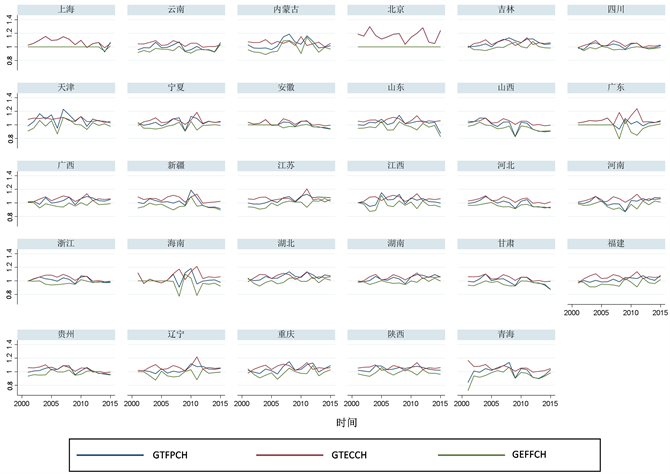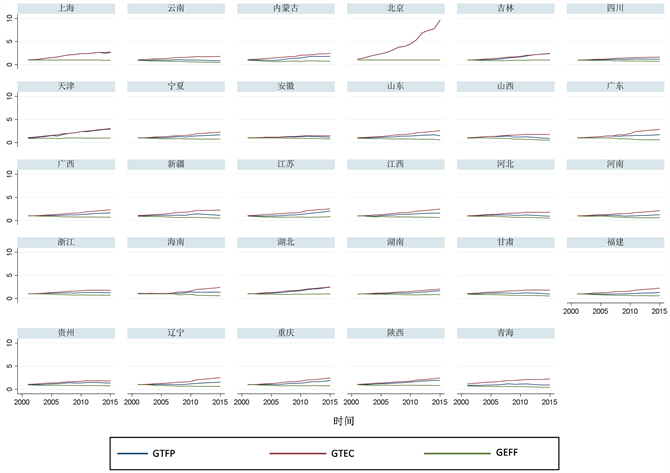# 中国绿色全要素生产率估算及时空演化分析An Estimation and Spatiotemporal Evolution Analysis of Green Total Factor Productivity in China

• 全文下载: PDF(1019KB)    PP.25-33   DOI: 10.12677/JLCE.2020.91004
• 下载量: 119  浏览量: 314

The energy consumption and environmental pollution were taken into the input-output analysis framework and the green total factor productivity and its decomposition of Chinese provinces were estimated by using DEA-Malmquist index analysis method based on 2000 - 2015 panel data of 29 provinces and cities in China. Then, the development trend and heterogeneity of green total factor productivity in different provinces and cities from the perspective of spatio-temporal evolution were analyzed. The results show that the improvement of China’s green total factor productivity depends on the progress of green technology to a large extent, and there are differences between coastal areas and inland areas. Therefore, different policies and measures should be taken to tap the potential of sustainable economic development when promoting sustainable economic and social development.

1. 引言

2. 研究方法

$\begin{array}{l}\mathrm{min}\left[\theta -\epsilon \left({\stackrel{^}{e}}^{\text{T}}{s}^{-}+{e}^{\text{T}}{s}^{+}\right)\right];\\ \text{s}\text{.t}\text{.}\text{\hspace{0.17em}}\underset{j=1}{\overset{k}{\sum }}{x}_{jk}{\lambda }_{j}+{s}^{-}=\theta {x}_{l}^{n};\underset{j=1}{\overset{k}{\sum }}{y}_{jm}{\lambda }_{j}-{s}^{+}={y}_{m}^{n};\\ \text{\hspace{0.17em}}\text{\hspace{0.17em}}\text{\hspace{0.17em}}\text{\hspace{0.17em}}\text{\hspace{0.17em}}{\lambda }_{j},{s}^{-},{s}^{+}\ge 0,n=1,2,\cdots ,K\end{array}$ (1)

DEA-Malmquist指数模型最早在1953年由瑞典的经济学家和统计学家Malmquist提出，用来对动态效率的变化趋势进行分析。目前，由Fare提出的DEA-Malmquist指数模型 ，是国内外学者们最常使用的测算模型。它假定在t时期的技术条件下，从t到t + 1时刻的DEA-Malmquist全要素生产率指数模型，如公式(2)所示：

${M}_{0}^{t}\left({x}^{t},{y}^{t}\right)=\frac{{D}_{0}^{t}\left({x}^{t+1},{y}^{t+1}\right)}{{D}_{0}^{t}\left({x}^{t},{y}^{t}\right)}$ (2)

${M}_{0}^{t+\text{1}}\left({x}^{t},{y}^{t}\right)=\frac{{D}_{0}^{t+\text{1}}\left({x}^{t+1},{y}^{t+1}\right)}{{D}_{0}^{t+\text{1}}\left({x}^{t},{y}^{t}\right)}$ (3)

${M}_{0}\left({x}^{t+1},{y}^{t+1},{x}^{t},{y}^{t}\right)={\left[\frac{{D}_{0}^{t}\left({x}^{t+1},{y}^{t+\text{1}}\right)}{{D}_{0}^{t}\left({x}^{t},{y}^{t}\right)}\cdot \frac{{D}_{0}^{t+1}\left({x}^{t+1},{y}^{t+\text{1}}\right)}{{D}_{0}^{t+\text{1}}\left({x}^{t},{y}^{t}\right)}\right]}^{1/2}$ (4)

DEA-Malmquist全要素生产率指数大于1，意味着全要素生产率增长，反之则意味着下降。DEA-Malmquist全要素生产率指数可进一步分解被分解，如公式(5)所示：

$\begin{array}{c}{M}_{0}\left({x}^{t+1},{y}^{t+1},{x}^{t},{y}^{t}\right)=\frac{{D}_{0}^{t+1}\left({x}^{t+1},{y}^{t+1}\right)}{{D}_{0}^{t}\left({x}^{t},{y}^{t}\right)}\cdot {\left[\frac{{D}_{0}^{t}\left({x}^{t+1},{y}^{t+\text{1}}\right)}{{D}_{0}^{t+\text{1}}\left({x}^{t+\text{1}},{y}^{t+\text{1}}\right)}\cdot \frac{{D}_{0}^{t}\left({x}^{t},{y}^{t}\right)}{{D}_{0}^{t+\text{1}}\left({x}^{t},{y}^{t}\right)}\right]}^{1/2}\\ ={\text{EFFCH}}_{t}^{t+1}\cdot {\text{TECHCH}}_{t}^{t+1}\end{array}$ (5)

3. 绿色全要素生产率估算

3.1. 变量选择及数据来源Table 1. Overview of input-output variables

3.2. 统计性描述Table 2. Statistical description of input-output variables

3.3. 绿色全要素生产率估算结果Figure 1. Overview of green total factor productivity related indexes in Chinese provincesFigure 2. Overview of green total factor productivity in Chinese provinces

4. 绿色全要素生产率时空演化分析

4.1. 绿色全要素生产率时间演化态势分析Table 3. Overview of national green total factor productivity from 2000 to 2015

4.2. 绿色全要素生产率区域对比分析Table 4. Overview of green total factor productivity in coastal areasTable 5. Overview of green total factor productivity in inland regions

5. 结论

  Solow, R.M. (1957) Technical Change and the Aggregate Production Function. Review of Economics and Statistics, 39, 312-320. https://doi.org/10.2307/1926047  Aigner, D.J. and Chu, S.F. (1968) On Estimating the Industry Production Function. American Economic Review, 58, 826-839.  杨汝岱. 中国制造业企业全要素生产率研究[J]. 经济研究, 2015, 50(2): 61-74.  余泳泽. 中国省际全要素生产率动态空间收敛性研究[J]. 世界经济, 2015, 38(10): 30-55.  Ahmed, E.M. (2012) Green TFP Intensity Impact on Sustainable East Asian Productivity Growth. Economic Analysis ＆ Policy, 42, 67-78. https://doi.org/10.1016/S0313-5926(12)50005-6  岳鸿飞, 徐颖, 周静. 中国工业绿色全要素生产率及技术创新贡献测评[J]. 上海经济研究, 2018(4): 52-61.  岳会, 于法稳. 中国棉花绿色全要素生产率及影响因素分析——基于Malmquist-Luenberger指数分析[J]. 价格理论与实践, 2020: 1-5.  Farrell, M.J. (1957) The Measurement of Productive Efficiency. Journal of the Royal Statistical Society, Series A (General), 120, 253-290. https://doi.org/10.2307/2343100  Fare, R., Grosskopf, S., Lindgren, B., et al. (1992) Productivity Changes in Swedish Pharmacies 1980-1989: A Non-Parametric Malmquist Approach. Journal of Productivity Analysis, 3, 81-97. https://doi.org/10.1007/978-94-017-1923-0_6  张军, 吴桂英, 张吉鹏. 中国省际物质资本存量估算: 1952-2000[J]. 经济研究, 2004(10): 35-44.  邱斌, 杨帅, 辛培江. FDI技术溢出渠道与中国制造业生产率增长研究: 基于面板数据的分析[J]. 世界经济, 2008(8): 20-31.